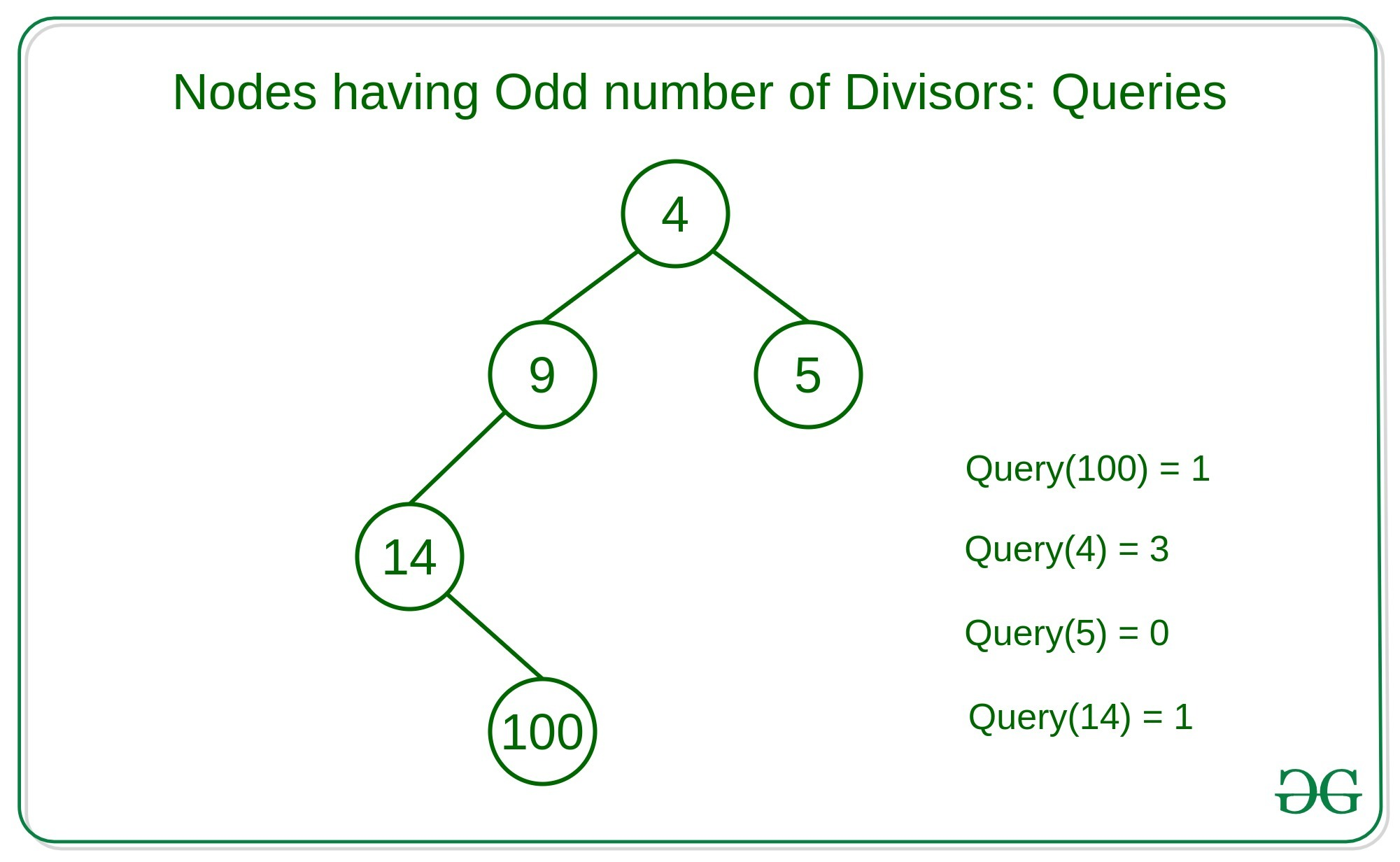# Count of nodes having odd divisors in the given subtree for Q queries

Given a binary tree and Q queries where each query contains a node of the binary tree, the task is to count the number of nodes which have an odd number of divisors in the subtree for Q queries.
Examples:

Input:Output: 1 3 0 1
Explanation:
Query 1: In the subtree rooted at node 100, there is only one node which is 100 which have 9 divisors {1, 2, 4, 5, 10, 20, 25, 50, 100}. Therefore, there is only one node having odd number of divisors.
Query 2: In the subtree rooted at node 4, there are 5 nodes out of which 3 nodes are having an odd number of divisors. That is {4, 9, 100}
Query 3: In the subtree rooted at node 5, there is only one node which is 5 which has two divisors. Therefore, there zero nodes having an odd number of divisors.

Naive Approach: A simple solution is to traverse the subtree for each query and find the count of nodes that are having an odd number of divisors.

Efficient Approach: The idea is to pre-compute the count of an odd number of divisors for each subtree and storing the count in hash-map. To pre-compute the count of nodes having an odd number of divisors we can use Depth First Search Traversal. Finally to check that the current node is having an odd number of divisors or not we can use the fact that every perfect square number has an odd number of divisors.

Below is the implementation of the above approach:

## C++

 `// C++ implementation to count the  ` `// number of nodes havving odd  ` `// number of divisors for each query ` ` `  `#include ` `using` `namespace` `std; ` ` `  `#define N 100001 ` ` `  `// Adjacency list  ` `// for tree. ` `vector<``int``> adj[N]; ` ` `  `// Array for values and  ` `// answer at ith node. ` `int` `a[N], ans[N]; ` ` `  `// Function to check whether N  ` `// has odd divisors or not ` `bool` `hasOddNumberOfDivisors(``int` `n) ` `{ ` `    ``if` `((``double``)``sqrt``(n) == (``int``)``sqrt``(n)) ` `        ``return` `true``; ` `    ``return` `false``; ` `} ` ` `  `// DFS function to pre-compute  ` `// the answers ` `int` `dfs(``int` `node, ``int` `parent) ` `{ ` `    ``// Initialize the count ` `    ``int` `count = 0; ` `    ``for` `(``auto` `i = adj[node].begin();  ` `        ``i != adj[node].end(); ++i) { ` `        ``if` `(*i != parent) { ` `             `  `            ``// Repeat for every child ` `            ``count += dfs(*i, node); ` `        ``} ` `    ``} ` `     `  `    ``// Increase the count if current node ` `    ``// has odd number of divisors ` `    ``if` `(hasOddNumberOfDivisors(a[node])) ` `        ``++count; ` ` `  `    ``ans[node] = count; ` `    ``return` `count; ` `} ` ` `  `// Driver Code ` `int` `main() ` `{ ` ` `  `    ``int` `n = 5, u, v, i, j; ` `    ``vector<``int``> q = {4, 1, 5, 3}; ` `     `  `    ``// Adjacency List ` `    ``adj.push_back(2); ` `    ``adj.push_back(1); ` `    ``adj.push_back(3); ` `    ``adj.push_back(2); ` `    ``adj.push_back(4); ` `    ``adj.push_back(3); ` `    ``adj.push_back(5); ` `    ``adj.push_back(1); ` `     `  `    ``a = 4; a = 9; ` `    ``a = 14; a = 100; ` `    ``a = 5; ` ` `  `    ``// Function call ` `    ``dfs(1, -1); ` ` `  `    ``for``(``int` `i = 0; i < q.size(); i++){ ` `        ``cout << ans[q[i]] << ``" "``; ` `    ``} ` `    ``return` `0; ` `} `

Output:

```1 3 0 1
```My Personal Notes arrow_drop_upCheck out this Author's contributed articles.

If you like GeeksforGeeks and would like to contribute, you can also write an article using contribute.geeksforgeeks.org or mail your article to contribute@geeksforgeeks.org. See your article appearing on the GeeksforGeeks main page and help other Geeks.

Please Improve this article if you find anything incorrect by clicking on the "Improve Article" button below.